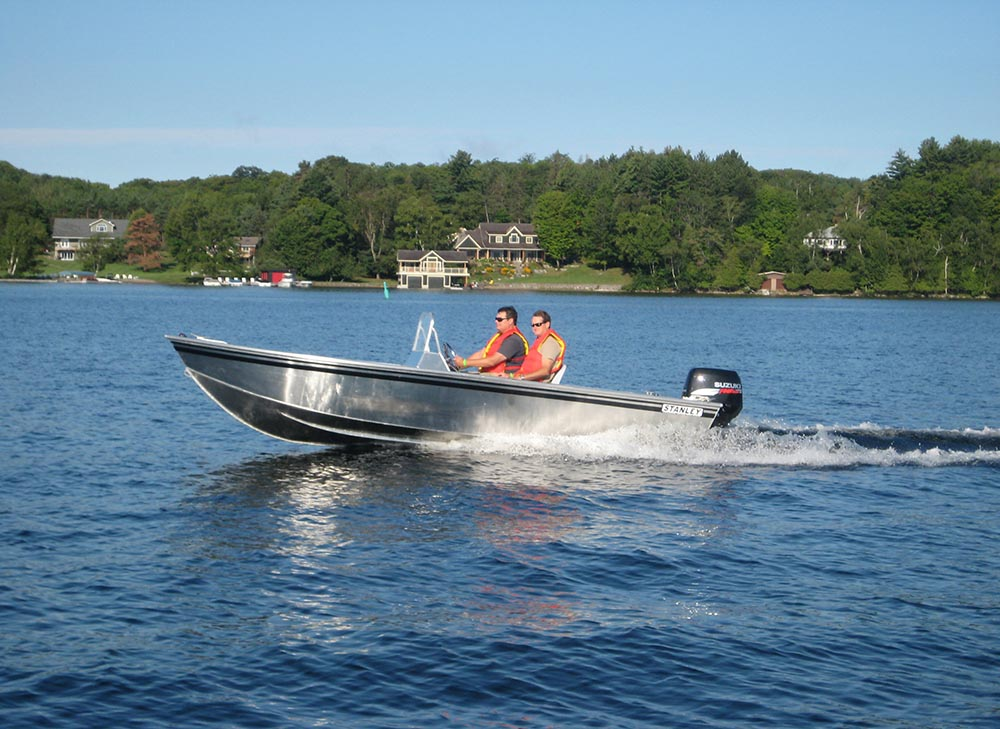# STANLEY TILLER

Regardless of your age, the Tiller will last a lifetime. Ideal for the cottage or camp, this all aluminum welded construction is what makes the Tiller the solid choice. Your grandchild will drive this boat one day – choose the Tiller.

•# Buy a TILLER

Find your local, approved Stanley Boat dealer.

# Standard Features

Models available in various lengths and configurations.

 3/16 inch hull 3/16 inch sides 1/8 inch sides v bottom floor lifting strakes welded gunwales keel guard 14′ 16′ 16′ W 16 WSC 16′ WDC 17″ 17′ SC 17′ DC X X X X X X X X X X X X X X X X X X X X X X X X X X X X X X X X X X X X X X X X X X X X X X X X X X X X X X X X X X X X X X X X X X X X X X X X X X X X X X X X X X X X X X X X X X X X X X X X X X X X X X X X X X X X X X X X

# Optional Features

These features may be available on your Tiller depending on the configuration.